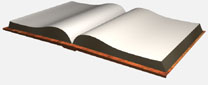# C-BOT

The following LCD screens provide the object target information.

We begin with Confidence. If the camera does not see a predetermined amount of target colored pixels, it assumes target is lost and if it’s above the amount target is acquired, see figure 18.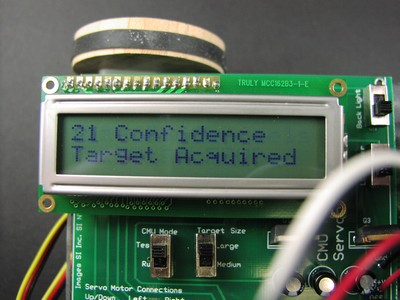Figure 18

When target is acquired the program will continue to map the target within its Field of View (FOV).

The next value after Confidence is the horizontal X.  The value of X determines whether the target is to the left, right or approximate center, see figure 19.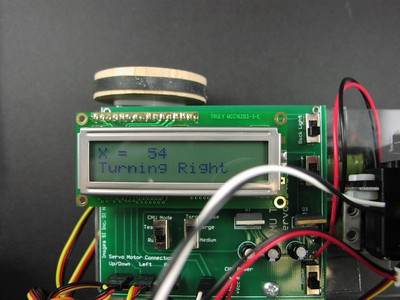Figure 19

The next value after the horizontal X is the vertical Y.  The value of Y determines whether the target is up, down or approximate center, see figure 20.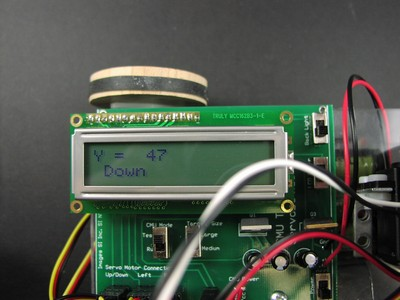Figure 20

The next value after the vertical Y is the number of pixels (size).  The number of pixels is used to determine distance from the camera (Z). If the pixels are under a certain value it is assume the target has moved away. If the pixel are above a certain value it is assumed the target is too close. In between these two values the object is in range, see figure 21.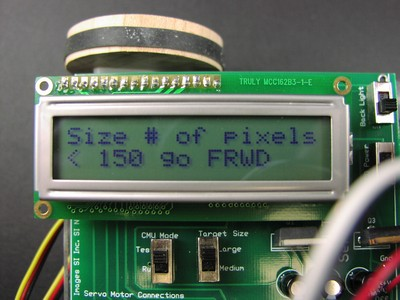Figure 21